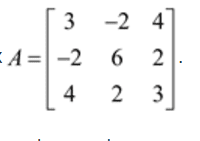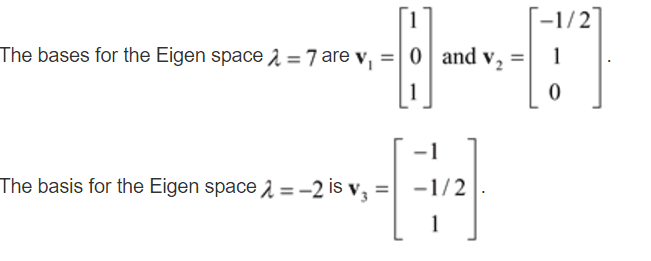# Extremely confused on finding eigenvectors

Jorge Cantu
Extremely confused on finding eigenvectors? Below I have a picture that gives the matrice and the eigenvectors. How did the solution find these eigenvectors??
i.e. the eigenvalues are 7 and -2http://tinypic.com/r/2liii68/9
http://tinypic.com/view.php?pic=2liii68&s=9#.VkY_YfmrSUk

Last edited by a moderator:

Homework Helper
an eigenvalue for a matrix A is a number c such that the matrix A-cI is singular. So to find them we set the determinant of that matrix equal to zero and solve the resulting cubic equation if we can. Then afterwards, we have the numbers c that work, and using each one in its turn we actually find the kernel vectors of the matrices A-cI. Remark: Since the matrix is symmetric about the main diagonal you are guranteed to have 3 independent eigenvectors, even if some of the eigenvalues are repeated. So you have to know how to take determinants, and then you have to know how to solve a homogeneous system.

Last edited by a moderator: